How to use this package (and why)

Introduction

The CFAcoop package equips you with functions to analyze data from the clonogenic assay (also called 'Colony Formation Assay') in presence or absence of cellular cooperation. Thus, this package allows you to robustly extract clonogenic survival information for your cell lines under a given treatment.

This vignette is meant to enable you to process your data following the method first presented in Brix et al., Radiation Oncology, 2020: "The clonogenic assay: robustness of plating efficiency-based analysis is strongly compromised by cellular cooperation". Therefore, the data presented in Figure 2 in Brix et al., which is provided within the package, is used to illustrate how to use the CFAcoop package. Further, it is shown, how the survival fractions of cooperative cell lines would look like, if cellular cooperation was ignored.

Cellular Cooperation

In order to avoid confusion, cellular cooperation should be defined. Understanding this concept comes easy, when starting from the opposite. Clonogenic growth of non-cooperative cells is independent of cell density. Thus, there is a constant relationship between cells seeded $$S$$ and colonies counted $$C$$: $C = a \cdot S,$ where $$a$$ corresponds just to the conventional plating efficiency ($$PE = \frac{C}{S}$$).

Now, cellular cooperation refers to the benefit cells can have from their surrounding neighbors which results in a non-constant relation of cells seeded and colonies counted (For details, see Brix et al., Radiation Oncology, 2020). The probability of clonogenic growth for a single cell increases with the number of surrounding cells to cooperate with. It has turned out that generalizing the equation above by a parameter $$b$$ adequately models the colonies counted of cooperative and non-cooperative cell lines: $C = a \cdot S^b.$ In this model, $$b = 1$$ gives the non-cooperative case and $$b > 1$$ corresponds to cooperative growth.

In short, a cell line is called cooperative, if $$b > 1$$.

Clonogenic Survival

Conventionally, clonogenic survival at a given treatment $$x$$ was determined as the ratio of colonies counted $$C_x$$ and the cells seeded $$S_x$$ scaled to the plating efficiency of a reference $$PE_0 = \frac{C_0}{S_0}$$ $SF'_x = \frac{\frac{C_x}{S_x}}{PE_0}.$

The new method now shifts the focus of the survival fraction directly to the number of cells needed to be seeded under the two conditions (treated and untreated) in order to achieve an identical expectation of the number of colonies formed $$C$$. Essentially, the new method does not focus on the number of colonies formed after growth in different cell densities, but on the number of seeded single cells with clonogenic potential before growing to identical colony numbers.

$SF_x(C) = \frac{S_0(C)}{S_x(C)} = exp\left( \frac{log\left(\frac{C}{a_0}\right)}{b_0} - \frac{log\left(\frac{C}{a_x}\right)}{b_x}\right)$

Obviously, for $$b_x = b_0 = 1$$ the equivalence $$SF_x(C) \equiv SF'$$ holds for all $$C$$, and thus, the non-cooperative case is well covered by the new method. Importantly, the conventional determination of clonogenic survival is heavily compromised by cellular cooperation, if present.

Getting Started

The data as presented in Figure 2 in Brix et al. is included in the package in form of a data.frame CFAdata. It can be loaded and summarized by:

library(CFAcoop)
#>      cell.line   Cells seeded           0 Gy             1 Gy
#>  T47D     :54   Min.   :   14.68   Min.   :  0.00   Min.   :  0.00
#>  MDA-MB231:84   1st Qu.:  146.78   1st Qu.:  8.00   1st Qu.: 10.00
#>  A549     :72   Median : 1000.00   Median : 35.50   Median : 35.00
#>  HCC1806  :48   Mean   : 3980.14   Mean   : 79.55   Mean   : 83.97
#>  SKBR3    :80   3rd Qu.: 4641.59   3rd Qu.:114.00   3rd Qu.:125.50
#>  SKLU1    :56   Max.   :31622.78   Max.   :535.00   Max.   :510.00
#>  BT20     :60                      NA's   :214      NA's   :214
#>       2 Gy             4 Gy             6 Gy             8 Gy
#>  Min.   :  0.00   Min.   :  0.00   Min.   :  0.00   Min.   :  0.00
#>  1st Qu.: 10.00   1st Qu.:  7.50   1st Qu.:  4.00   1st Qu.:  1.00
#>  Median : 35.00   Median : 29.00   Median : 19.00   Median :  7.00
#>  Mean   : 85.31   Mean   : 70.01   Mean   : 56.17   Mean   : 30.21
#>  3rd Qu.:119.00   3rd Qu.:105.00   3rd Qu.: 73.25   3rd Qu.: 30.00
#>  Max.   :480.00   Max.   :400.00   Max.   :450.00   Max.   :250.00
#>  NA's   :219      NA's   :215      NA's   :214      NA's   :213

Fast Analysis and Plotting of Results

The shortcut to analyze data, is using the wrapper function analyze_survival(RD, name, xtreat) where RD is a data.frame or matrix containing your numbers of seeded cells (first column) and numbers of colonies counted under the treatments (numeric argument, e.g. the dose applied xtreat = c(0,1,2,4,6,8)).

The returned objects should be concatenated in a list-object and can be plotted by plot_sf().

data1 <- subset.data.frame(x = CFAdata, subset = CFAdata$cell.line == "T47D") data2 <- subset.data.frame(x = CFAdata, subset = CFAdata$cell.line == "BT20")
SF <- vector("list", 2)
SF[] <- analyze_survival(
RD = data1[, c("Cells seeded","0 Gy","1 Gy","2 Gy","4 Gy","6 Gy","8 Gy")],
name = as.character(data1[1,1]),
xtreat = c(0, 1, 2, 4, 6, 8),
c_range = c(5, 20, 100))
SF[] <- analyze_survival(
RD = data2[,-1],
name = as.character(data2[1,1]),
xtreat = c(0, 1, 2, 4, 6, 8))
plot_sf(SF = SF)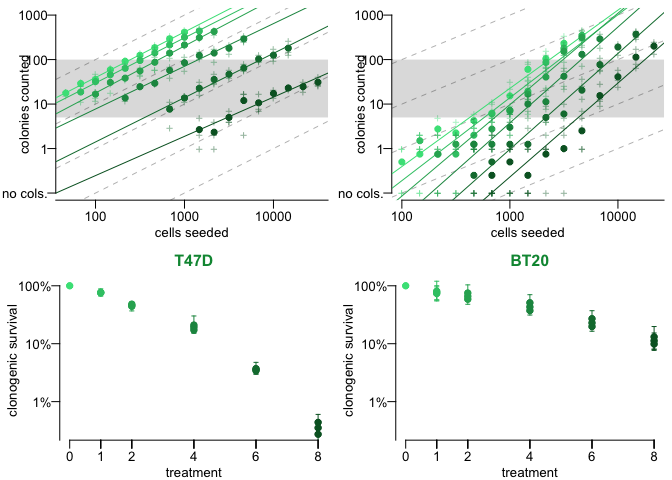Raw data from single replicates is plotted as '$$+$$'-symbols. Corresponding regression lines are calculated using the mean values of replicates of identical numbers of cells seeded, which are plotted as dots. The color indicates the treatment (irradiation with 0 to 8 Gy) and links the numbers of colonies counted from the upper plot to the calculated clonogenic survival in the lower plot. Shaded areas indicate the span from $$C = 5$$ to $$C = 100$$, which is within the target region of good experimental practice. Dashed lines show regression lines with a slope of $$b=1$$ (at $$log10(a)$$ varying from 0 to 4) for orientation, so that any substantially non-linear relation (i.e. $$b \neq 1$$) between the number of colonies counted ($$C$$) and the number of cells seeded ($$S$$) can be spotted easily.
The dots in the treatment response curves correspond to the survival fractions at $$C = 5, 20$$ and $$100$$, with error bars indicating the uncertainty bands calculated as $$2.5\%$$ and $$97.5\%$$-percentiles of the parametric bootstrap ($$N = 1000$$) evaluating the covariance matrices of the power regressions.

All information used for plotting is contained in the objects returned by analyze_survival.

A data.frame with a summary of the estimated survival fractions can be generated by

summary_df <- export_sf(SF)

to export this data.frame in a csv-File, execute:  write.csv(x = summary_df,file = "CFAcoopResult.csv")

The data.frame includes the following columns

colnames(summary_df)
#>   "cell.line"         "treatment"         "ln.a."
#>   "ln.a..se"          "b"                 "b.se"
#>   "r"                 "SF(5)"             "SF(5)_uncert_lb"
#>  "SF(5)_uncert_ub"   "SF(20)"            "SF(20)_uncert_lb"
#>  "SF(20)_uncert_ub"  "SF(100)"           "SF(100)_uncert_lb"
#>  "SF(100)_uncert_ub"
#>   cell.line treatment  ln.a. ln.a..se     b  b.se      r  SF(5) SF(5)_uncert_lb
#> 1      T47D         0 -1.040    0.108 1.024 0.020 -0.983     NA              NA
#> 2      T47D         1 -1.277    0.094 1.015 0.016 -0.986 0.7726          0.6621
#> 3      T47D         2 -1.984    0.163 1.055 0.026 -0.987 0.4414          0.3682
#> 4      T47D         4 -2.375    0.377 0.960 0.054 -0.990 0.2095          0.1553
#> 5      T47D         6 -4.247    0.359 0.996 0.044 -0.993 0.0372          0.0296
#> 6      T47D         8 -5.460    0.873 0.882 0.098 -0.994 0.0044          0.0034
#>   SF(5)_uncert_ub SF(20) SF(20)_uncert_lb SF(20)_uncert_ub SF(100)
#> 1              NA     NA               NA               NA      NA
#> 2          0.8872 0.7630           0.6966           0.8313  0.7519
#> 3          0.5317 0.4595           0.4109           0.5131  0.4815
#> 4          0.3012 0.1914           0.1602           0.2325  0.1724
#> 5          0.0476 0.0358           0.0314           0.0405  0.0343
#> 6          0.0060 0.0035           0.0026           0.0045  0.0027
#>   SF(100)_uncert_lb SF(100)_uncert_ub
#> 1                NA                NA
#> 2            0.7168            0.7868
#> 3            0.4529            0.5115
#> 4            0.1512            0.1934
#> 5            0.0303            0.0386
#> 6            0.0012            0.0044
summary(summary_df)
#>   cell.line           treatment       ln.a.            ln.a..se
#>  Length:12          Min.   :0.0   Min.   :-16.049   Min.   :0.0940
#>  Class :character   1st Qu.:1.0   1st Qu.:-12.333   1st Qu.:0.3100
#>  Mode  :character   Median :3.0   Median : -7.246   Median :0.8135
#>                     Mean   :3.5   Mean   : -7.676   Mean   :0.7391
#>                     3rd Qu.:6.0   3rd Qu.: -2.277   3rd Qu.:1.0542
#>                     Max.   :8.0   Max.   : -1.040   Max.   :1.7440
#>
#>        b              b.se               r               SF(5)
#>  Min.   :0.882   Min.   :0.01600   Min.   :-0.9940   Min.   :0.0044
#>  1st Qu.:1.010   1st Qu.:0.03950   1st Qu.:-0.9915   1st Qu.:0.1234
#>  Median :1.407   Median :0.10450   Median :-0.9900   Median :0.2912
#>  Mean   :1.502   Mean   :0.09942   Mean   :-0.9891   Mean   :0.3454
#>  3rd Qu.:2.069   3rd Qu.:0.14800   3rd Qu.:-0.9868   3rd Qu.:0.5498
#>  Max.   :2.155   Max.   :0.21000   Max.   :-0.9830   Max.   :0.7726
#>                                                      NA's   :2
#>  SF(5)_uncert_lb   SF(5)_uncert_ub      SF(20)       SF(20)_uncert_lb
#>  Min.   :0.00340   Min.   :0.0060   Min.   :0.0035   Min.   :0.0026
#>  1st Qu.:0.09635   1st Qu.:0.1560   1st Qu.:0.1322   1st Qu.:0.1002
#>  Median :0.23830   Median :0.3800   Median :0.3296   Median :0.2737
#>  Mean   :0.28139   Mean   :0.4285   Mean   :0.3649   Mean   :0.3039
#>  3rd Qu.:0.45138   3rd Qu.:0.6786   3rd Qu.:0.6074   3rd Qu.:0.5038
#>  Max.   :0.66210   Max.   :0.9580   Max.   :0.7673   Max.   :0.6966
#>  NA's   :2         NA's   :2        NA's   :2        NA's   :2
#>  SF(20)_uncert_ub    SF(100)       SF(100)_uncert_lb SF(100)_uncert_ub
#>  Min.   :0.0045   Min.   :0.0027   Min.   :0.00120   Min.   :0.0044
#>  1st Qu.:0.1731   1st Qu.:0.1414   1st Qu.:0.09825   1st Qu.:0.1945
#>  Median :0.3970   Median :0.3759   Median :0.29410   Median :0.4415
#>  Mean   :0.4417   Mean   :0.3911   Mean   :0.31190   Mean   :0.5041
#>  3rd Qu.:0.7525   3rd Qu.:0.6895   3rd Qu.:0.52302   3rd Qu.:0.7655
#>  Max.   :1.0034   Max.   :0.8082   Max.   :0.71680   Max.   :1.1983
#>  NA's   :2        NA's   :2        NA's   :2         NA's   :2

All information of this data.frame is also accessible directly in the object returned by analyze_survival. For instance, the information about the regression of the 0 Gy reference of the cell line BT20 or the survival fractions of the 5 treatments for T47D (at $$C = 5, 20$$ and $$100$$) can be recalled by:

SF[]$fit #> [] #> #> Call: #> lm(formula = "lnC ~ 1 + lnS", data = x) #> #> Residuals: #> Min 1Q Median 3Q Max #> -0.10193 -0.02679 -0.01385 0.04023 0.08414 #> #> Coefficients: #> Estimate Std. Error t value Pr(>|t|) #> (Intercept) -1.03970 0.10833 -9.598 2.80e-05 *** #> lnS 1.02403 0.01983 51.645 2.67e-10 *** #> --- #> Signif. codes: 0 '***' 0.001 '**' 0.01 '*' 0.05 '.' 0.1 ' ' 1 #> #> Residual standard error: 0.05894 on 7 degrees of freedom #> Multiple R-squared: 0.9974, Adjusted R-squared: 0.997 #> F-statistic: 2667 on 1 and 7 DF, p-value: 2.673e-10 SF[]$SF
#> []
#>         5        20       100
#> 0.7337435 0.7672861 0.8081559
#>
#> []
#>         5        20       100
#> 0.5860258 0.6566601 0.7494108
#>
#> []
#>         5        20       100
#> 0.3727500 0.4308404 0.5097292
#>
#> []
#>         5        20       100
#> 0.1976257 0.2284513 0.2703190
#>
#> []
#>          5         20        100
#> 0.09867994 0.11254453 0.13110228

Details for Focussed Analysis

Assess Cellular Cooperation

Key to the robust analysis of clonogenic analysis data is the modeling of the cellular cooperation. We assume that the underlying functional dependency of seeded cells and counted colonies is of the form $C = a \cdot S^{b},$ where $$b$$ indicates the degree of cellular cooperation ($$b = 1$$ is implicitely assumed for the PE-based approach).

The coefficient $$b$$ is estimated in a linear regression model $log(C) = log(a) + b \cdot log(S) + \varepsilon, \varepsilon \sim \mathcal{N}(0,\sigma^2).$

The function pwr_reg(seede, counted) provides this regression and returns a summary.lm object.

Note that the analysis of cellular cooperation is restricted to the range of seeded cells, where at least one colony was observed. Outside this range, the attempt of studying clonogenic survival based on no observed colony counts is not reasonable and thus, pwr_reg will remove those data points from analysis. Thus, it is strongly recommended to use the averaged data for regression. By doing so, the range of the independent variable of the regression is widened. (Removing only those replicates with no colonies at one or few specific cell densities would bias the model fitting.)

data <- subset.data.frame(x = CFAdata, subset = CFAdata$cell.line == "BT20") data <- aggregate(x = data[, -1], by = list(data$Cells seeded), FUN = "mean", na.rm = TRUE)
par_0 <- pwr_reg(seeded = data$Cells seeded, counted = data$0 Gy)
par_0$coefficients #> Estimate Std. Error t value Pr(>|t|) #> (Intercept) -9.031773 0.7538225 -11.98130 2.169497e-06 #> lnS 1.758593 0.1172839 14.99433 3.864744e-07 plot(x = log10(data$Cells seeded), y = log10(data$0 Gy),xlim = c(2,3.5)) abline(a = log10(exp(1)) * par_0$coefficients[1, 1], b = par_0$coefficients[2, 1])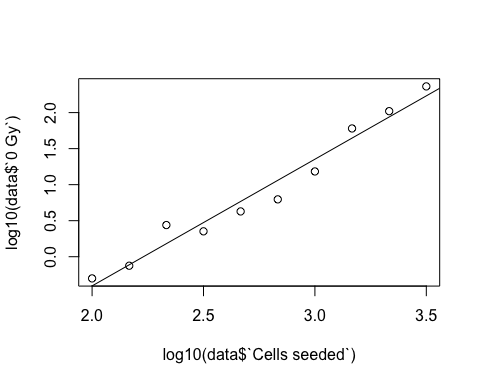With the results of this function, we can also test for cellular cooperation. Note, that the p-value in the coefficients table corresponds to the null hypothesis $$b = 0$$, but we are interested in the null hypothesis of $$b \leq 1$$. Thus, we find our p-value of interest by computing p_value <- (1 - pt( q = (par_0$coefficients[2, 1] - 1) / par_0$coefficients[2, 2], df = par_0$df
))

Thus, BT20 is higly cooperative ($$b = 1.76$$, $$\hat{\sigma}_b = 0.12$$, $$p < 0.001$$).

Determine Clonogenic Survival Fractions

In this package, the survival fraction $$SF(C)$$ for clonogenic survival is calculated as the number of cells that need to be seeded without treatment divided by the number of cells needed to be seeded with treatment for obtaining the same expectation of colonies counted $$C$$. $SF(C) = \frac{S_0(C)}{S_x(C)} = exp\left( \frac{log\left(\frac{C}{a_0}\right)}{b_0} - \frac{log\left(\frac{C}{a_x}\right)}{b_x} \right)$

Given two parameter sets of clonogenic assay data, the clonogenic survival can be calculated as:

data <- subset.data.frame(x = CFAdata, subset = CFAdata$cell.line == "BT20") data <- aggregate(x = data[, -1], by = list(data$Cells seeded), FUN = "mean", na.rm = TRUE)
par_0 <- pwr_reg(seeded = data$Cells seeded, counted = data$0 Gy)
par_4 <- pwr_reg(seeded = data$Cells seeded, counted = data$4 Gy)
calculate_sf(par_ref = par_0, par_treat = par_4, c_range = c(5, 100))
#>         5       100
#> 0.3727500 0.5097292

Since the SF-values are solely dependent on the estimated parameters in the power regression, the inherent uncertainty can be assessed via parametric bootstrapping, namely:

ParSet_0 <- mvtnorm::rmvnorm(n = 10^4,mean = par_0$coefficients[,1], sigma = vcov(object = par_0)) ParSet_4 <- mvtnorm::rmvnorm(n = 10^4,mean = par_4$coefficients[,1],
sigma = vcov(object = par_4))
parbootstrap <- calculate_sf(par_ref = ParSet_0,
par_treat = ParSet_4,
c_range = c(5, 100))
cat(c(mean(parbootstrap),sd(parbootstrap)))
#> 0.3756744 0.03958879
cat(quantile(x = parbootstrap,probs = c(0.025,0.5,0.975)))
#> 0.3043772 0.3733833 0.460289

Remark on Ignoring Cellular Cooperation

Note that in case of cooperative cell lines, the parameter $$a$$ does not correspond to a plating efficiency as for non-cooperative cell lines. The concept of a characteristic plating efficiency does not apply to cooperative cell lines.

What's the problem with PE-based analysis?

In short: The plating efficiency frequently is not as constant as it needs to be in order to serve as an adequate normalization factor.

To illustrate this, we compare the PE-based calculated $$SF'$$-values with the $$SF(C = 20)$$-values calculated with the new method for (1) the non-cooperative cell line T47D and (2) the cooperative cell line (BT20).

(1) The non-cooperative case

For calculating the survival fraction, plating efficiencies are required. Plating efficiencies ($$C/S$$) are calculated easily as:

data <- subset.data.frame(x = CFAdata, subset = CFAdata$cell.line == "T47D") data <- aggregate(x = data[, -1], by = list(data$Cells seeded), FUN = "mean", na.rm = TRUE)
PE_x <- data$4 Gy / data$Cells seeded
PE_0 <- data$0 Gy / data$Cells seeded
plot(x = rep(c(0, 1), each = 18), y = c(PE_0, PE_x), lty = 0, ylim = c(0,0.5),xlim = c(-0.1,1.1),
xlab = "treatment", ylab = "C / S", axes = FALSE, main = "T47D")
axis(side = 1,at = c(0,1),labels = c("0 Gy","4 Gy"))
axis(side = 2,at = seq(0,0.5,0.1))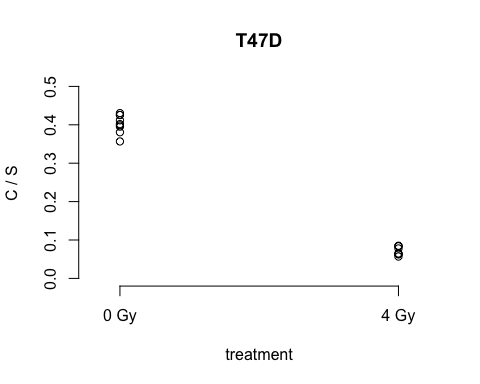Now, which values are to be compared?

When there is no effect of the cell density, as assumed by the conventional approach (not taking cellular cooperation into account), each combination (of a $$C_{0 Gy}/S_{0 Gy}$$- and $$C_{4 Gy}/S_{4 Gy}$$-value) is equally reliable.

Thus, the full set of all combinations is:

SF_resample <- rep(PE_x, each = length(PE_0)) / rep(PE_0, times = length(PE_x))
hist(SF_resample, breaks = 25,xlim = c(0.12,0.25),xlab = "(C_4/S_4) / (C_0/S_0)",
main = "valid PE-based SF'-values")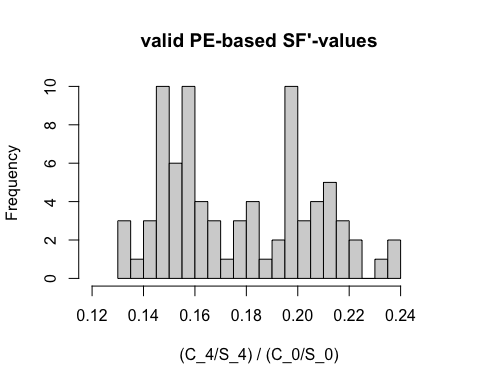Without the assessment of cellular cooperation, conventional calculation of an $$SF'$$-value, corresponds to picking randomly a sample from the distribution shown in the histogram above.

A comparison of the range of this distribution and the calculated uncertainties of the new method shows, that for non-cooperative cell lines such as T47D, there is no big difference in this variability/uncertainty.

range(SF_resample,na.rm = TRUE)
#>  0.1322039 0.2383178
as_nc_0 <- analyze_survival(RD = data[,-1],c_range = c(20))
as_nc_0$uncertainty[] #> [,1] [,2] #> 20 0.1610909 0.2363611 (2) The cooperative case Now, making the same comparison as in (1) for the cooperative cell line BT20 shows the disastrous effect of ignoring the coefficient $$b$$, when it is in fact different from $$1$$. data(CFAdata) data <- subset.data.frame(x = CFAdata, subset = CFAdata$cell.line == "BT20")
data <- aggregate(x = data[, -1], by = list(data$Cells seeded), FUN = "mean", na.rm = TRUE) PE_x <- data$4 Gy / data$Cells seeded PE_0 <- data$0 Gy / data$Cells seeded plot(x = rep(c(0, 1), each = length(PE_x)), y = c(PE_0, PE_x), lty = 0, ylim = c(0,0.08),xlim = c(-0.1,1.1), xlab = "treatment", ylab = "C / S", axes = FALSE, main = "BT20") axis(side = 1,at = c(0,1),labels = c("0 Gy","4 Gy")) axis(side = 2,at = seq(0,0.08,0.02),las = 1)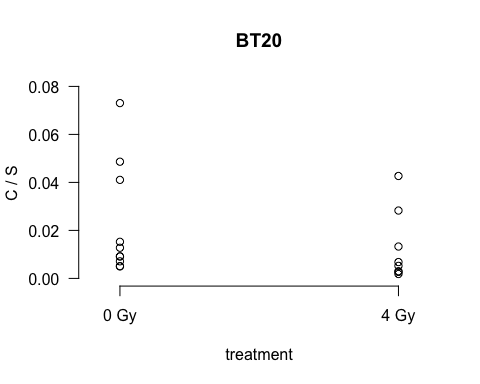SF_resample <- rep(PE_x, each = length(PE_0)) / rep(PE_0, times = length(PE_x)) hist(SF_resample, breaks = 100,xlim = c(0,10),xlab = "(C_4/S_4) / (C_0/S_0)", main = "valid PE-based SF'-values")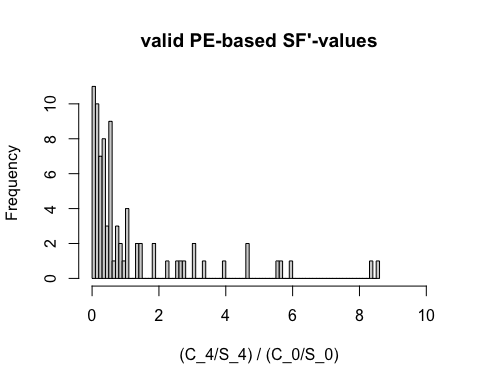The range of the PE-based $$SF'$$-values does not correspond to the uncertainty of the $$SF(20)$$-values: range(SF_resample,na.rm = TRUE) #>  0.02511682 8.53525274 as_c_4 <- analyze_survival(RD = data[,-1],c_range = c(20)) as_c_4$uncertainty[]
#>         [,1]     [,2]
#> 20 0.3557155 0.529127

Even though the survival fraction can be accurately estimated under consideration of cellular cooperation

sem_SF20 <- (as_c_4$uncertainty[]-as_c_4$uncertainty[])/4
cat("    SF(20) : ",round(as_c_4\$SF[],digits = 3),"\n")
#>     SF(20) :  0.431
cat("SEM SF(20) : ",round(sem_SF20,digits = 3),"\n")
#> SEM SF(20) :  0.043

the PE-based approach fails in returning a trustworthy estimate of the fraction of cells losing their potential due to the treatment (see histogram).

In particular, the average of PE-based $$SF'$$ calculations does not asymptotically tend to an interpretable value. In case of strong cellular cooperation, the PE-based calculated $$SF'$$-value is heavily affected by this cellular cooperation and the treatment effect of interest is degraded to a side effect:

cat("    SF'    : ",round(mean(SF_resample,na.rm = TRUE),digits = 3),"\n")
#>     SF'    :  1.242
cat("SEM SF'    : ",round(sd(SF_resample,na.rm = TRUE)/
sqrt(length(SF_resample)),digits = 3),"\n")
#> SEM SF'    :  0.122

As a consequence, the fraction of cells keeping their clonogenic potential after treatment is far outside the uncertainty interval of the average $$SF'$$-value of the PE-based approach.

Conclusion from (1) and (2)

Before calculating PE-based survival fractions, one must check whether there is cellular cooperation or not.

Essentially, to decide, whether you have a cooperativity issue or not, you need to conduct the same analysis and to generate the same data that is necessary to solve this issue anyways.